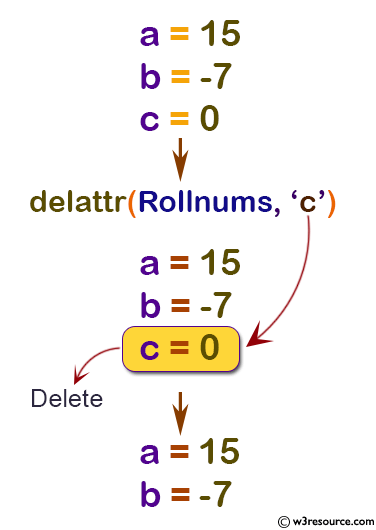# Python: delattr() function

## delattr() function

The delattr() function is used to delete the specified attribute from the specified object.

Version:

(Python 3)

Syntax:

```delattr(object, name)
```

Parameter:

Name Description Required /
Optional
object The object from which name attribute is to be removed. Required.
name The name of one of the object’s attributes. Required.

Return value:

The delattr() doesn't return any value (returns None).

Example: Python delattr() function

``````class Rollnums:
a = 15
b = -7
c = 0

rno = Rollnums()

print('a = ',rno.a)
print('b = ',rno.b)
print('c = ',rno.c)

delattr(Rollnums, 'c')

print('--After deleting c attribute--')
print('a = ',rno.a)
print('b = ',rno.b)``````

Output:

```a =  15
b =  -7
c =  0
--After deleting c attribute--
a =  15
b =  -7
```

Pictorial Presentation:Python Code Editor:

Previous: complex()
Next: dict()

Test your Python skills with w3resource's quiz

﻿

## Python: Tips of the Day

How do I check if a list is empty?

For example, if passed the following:

```a = []
if not a:
print("List is empty")
```

Ref: https://bit.ly/2A4JXx9